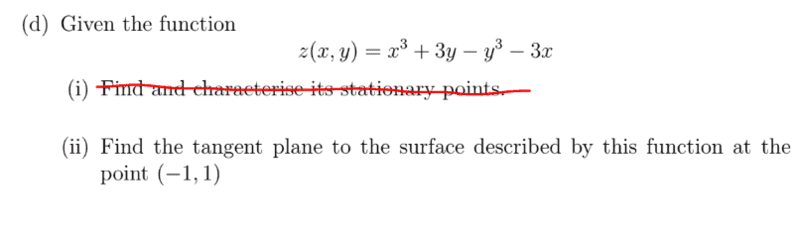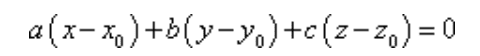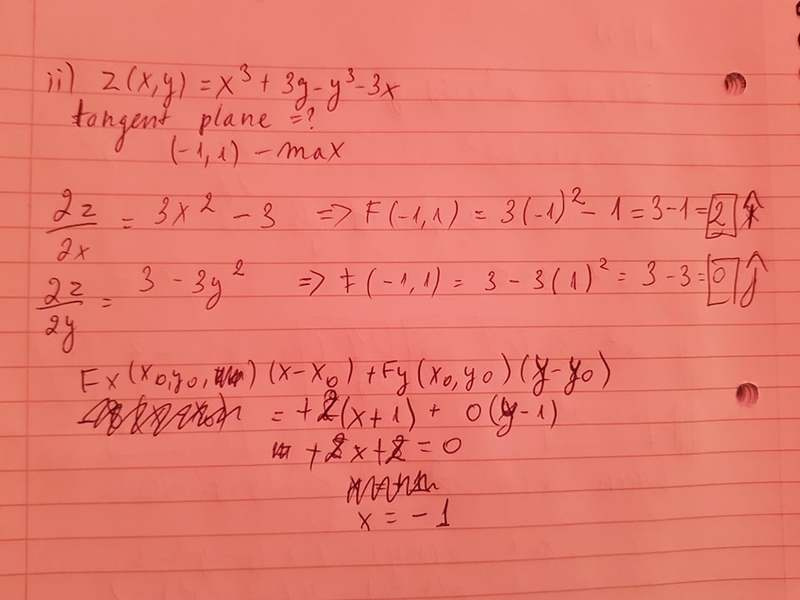# Find the tangent plane given at the stationary point.

• Jozefina Gramatikova

## Homework Statement## Homework Equations[/B]

## The Attempt at a SolutionI got -1, but the answer says "6". Could you help me, please?

#### Attachments

I see a mistake in the x-partial. You have not substituted in correctly.

I see a mistake in the x-partial. You have not substituted in correctly.
Oh, thanks! But then 3-3=0 and the whole thing is 0

So what do you think the tangent plane looks like? And what is its formula?

PS. This is all the help I can give, sorry.

Oh, thanks! But then 3-3=0 and the whole thing is 0
In the relevant equations, you have correctly given the equation of a plane. You can rewrite this as
##(a, b, c) \cdot (x-x_0,y-y_0,z-z_0) = 0##

This says that ##(a, b, c)## is normal to all of the vectors in the plane.

If this plane is tangent to the surface described by ##(x, y,f(x,y))## at ##(x_0,y_0,z_0)##, then ##(a, b, c)## would also have to be normal to that surface at that point. How would you find the vector ##(a, b, c)## that is normal to the surface?